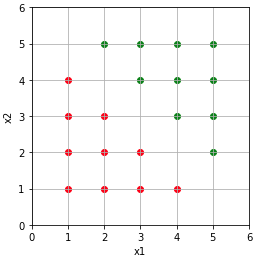A classifier is binary when it classifies data into one of 2 possible classes :

• 0 or negative samples
• 1 or positive samples

A classifier is linear when the samples are linearly seperable. It means that you can separate the positive and negative samples by a line.

Let’s say we have positive and negative examples, and we want to classify them.Each sample has two features x1 and x2, let’s note y the class of the sample, such as :

$$y \in \left \{ 0,1 \right \}$$ The red samples will be the negative ones, and the green ones will be the positive.

To classify them, the obvious thing to do is to seperate them by a line :Ok, nice, but how do we actually know that an example is a positive or a negative one ?

Weeeeellll, using maths !

Let’s say we have positive and negative examples seperate by the blue line of equation : $$x2 = -x1 + 6$$. Note that this line has a direction vector :

$\mathbf{d} = \begin{pmatrix}1\\-1\end{pmatrix}$

From this vector we can easily find a normal vector (red vector) :

$\mathbf{u} = \begin{pmatrix}1\\1\end{pmatrix}$

To make our life easier, we transform this vector into a unit vector :

$\mathbf{w} = \frac{\mathbf{u}}{\|\mathbf{u}\|}$ $\|\mathbf{w}\| = 1$We want to classify the purple sample. This sample can be represented by the green vector :

$\mathbf{x} = \begin{pmatrix}x1\\x2\end{pmatrix}$

To classify this sample, the idea is to project $$\mathbf{x}$$ (the green vector) on $$\mathbf{w}$$ (equivalent to the red vector) to obtain the solid black segment of length $$l$$ :

$l = \|\mathbf{x}\| \cdot \cos(\mathbf{w},\mathbf{x})$

From the dot product definition we know that :

$\mathbf{w} \cdot \mathbf{x} = \|\mathbf{w}\| \cdot \|\mathbf{x}\| \cdot \cos(\mathbf{w},\mathbf{x}) = \|\mathbf{w}\| \cdot l$

We can rewrite $$l$$ as :

$l = \frac{\mathbf{w} \cdot \mathbf{x}}{\| \mathbf{w} \|}$

Because $$\mathbf{w}$$ is a unit vector, we can finally write :

$l = \mathbf{w} \cdot \mathbf{x}$

To determine if an example is positive or negative, we just need to look if it’s bellow or above the line. So we just need to compare $$l$$ to the length of the red dashed line ($$b$$).

$\begin{cases} 1 & \text{ if } l\geq b \\ 0 & \text{ if } l < b \end{cases}$

or

$\begin{cases} 1 & \text{ if } l-b\geq0 \\ 0 & \text{ if } l-b < 0 \end{cases}$

To write this down in a single equation, we can use the “Heaviside step function” defined by :

$\mathcal{H}(t) = \begin{cases} 1 & \text{ if } t \geq 0 \\ 0 & \text{ if } t < 0 \end{cases}$If we replace $$t$$ by $$l-b$$ we obtain:

$y = \mathcal{H}(l-b)$

and with the complete formula:

$y = \mathcal{H}(\mathbf{x} \cdot \mathbf{w}-b)$

And there we go, we have a linear binary classifier.